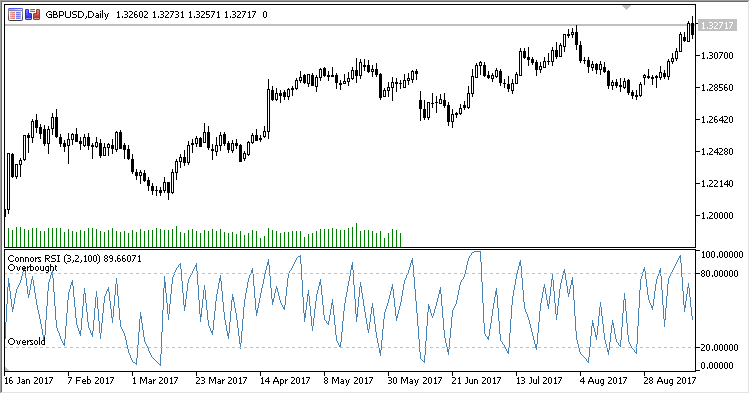Find us on Telegram!
Interesting script?
So post a link to it -
let others appraise it
You liked the script? Try it in the MetaTrader 5 terminal# Connors_RSI - indicator for MetaTrader 5

|
Views:
5413
Rating:
(13)
Published:
2018.10.25 17:33

Connors RSI is an RSI oscillator by Larry Connors.

It has six input parameters:

• RSI period - calculation period
• Pulse RCI period - impulse RSI period
• Percent rank period - range of calculating the change rate in %
• Applied price
• Overbought - overbought level
• Oversold - oversold level

Calculation:

```CRSI = (RSI1+RSI2+RSI3) / 3.0
```

where:

```RSI1 - RSI(Applied price,RSI period)
RSI2 - RSI(UpDown,Smoothing period)
RSI3 - 100.0 * Count / Percent rank period
UpDown - number of consecutive days closed above/below previous days:
If a day is closed above the past, a previous value is increased by +1,
If a day is closed below the past, a previous value is decreased by -1,
Otherwise:
if the previous value is positive, the current value is 1,
if the previous value is negative, the current value is -1,
Count - number of positive increments of a two-period RSI within the Percent rank period range
```Translated from Russian by MetaQuotes Ltd.
Original code: https://www.mql5.com/ru/code/22421Simple_Decycler

Simple Decycler indicatorDecycler_Oscillator

Decycler Oscillator indicatorGeneralized DEMA

Generalized DEMAAvg_MA

Average from MA indicator